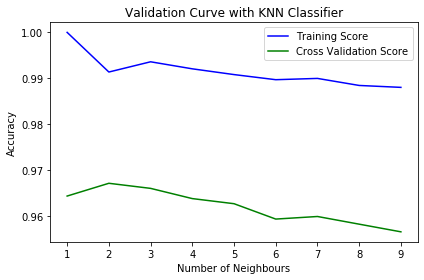Related Articles

# Validation Curve

• Last Updated : 22 Apr, 2021

A Validation Curve is an important diagnostic tool that shows the sensitivity between to changes in a Machine Learning model’s accuracy with change in some parameter of the model.
A validation curve is typically drawn between some parameter of the model and the model’s score. Two curves are present in a validation curve – one for the training set score and one for the cross-validation score. By default, the function for validation curve, present in the scikit-learn library performs 3-fold cross-validation.
A validation curve is used to evaluate an existing model based on hyper-parameters and is not used to tune a model. This is because, if we tune the model according to the validation score, the model may be biased towards the specific data against which the model is tuned; thereby, not being a good estimate of the generalization of the model.
Interpreting a Validation Curve
Interpreting the results of a validation curve can sometimes be tricky. Keep the following points in mind while looking at a validation curve :

• Ideally, we would want both the validation curve and the training curve to look as similar as possible.
• If both scores are low, the model is likely to be underfitting. This means either the model is too simple or it is informed by too few features. It could also be the case that the model is regularized too much.
• If the training curve reaches a high score relatively quickly and the validation curve is lagging behind, the model is overfitting. This means the model is very complex and there is too little data; or it could simply mean there is too little data.
• We would want the value of the parameter where the training and validation curves are closest to each other.

Implementation of Validation Curves in Python :
https://scikit-learn.org/stable/auto_examples/datasets/plot_digits_last_image
For this example, we will use k-Nearest Neighbour classifier and will plot the accuracy of the model on the training set score and the cross-validation score against the value of ‘k’, i.e., the number of neighbours to consider.
Code: Python code to implement 5-fold cross-validation and to test the value of ‘k’ from 1 to 10.

## python3

 `# Import Required libraries``import` `matplotlib.pyplot as plt``import` `numpy as np``from` `sklearn.datasets ``import` `load_digits``from` `sklearn.neighbors ``import` `KNeighborsClassifier``from` `sklearn.model_selection ``import` `validation_curve` `# Loading dataset``dataset ``=` `load_digits()` `# X contains the data and y contains the labels``X, y ``=` `dataset.data, dataset.target` `# Setting the range for the parameter (from 1 to 10)``parameter_range ``=` `np.arange(``1``, ``10``, ``1``)` `# Calculate accuracy on training and test set using the``# gamma parameter with 5-fold cross validation``train_score, test_score ``=` `validation_curve(KNeighborsClassifier(), X, y,``                                       ``param_name ``=` `"n_neighbors"``,``                                       ``param_range ``=` `parameter_range,``                                        ``cv ``=` `5``, scoring ``=` `"accuracy"``)` `# Calculating mean and standard deviation of training score``mean_train_score ``=` `np.mean(train_score, axis ``=` `1``)``std_train_score ``=` `np.std(train_score, axis ``=` `1``)` `# Calculating mean and standard deviation of testing score``mean_test_score ``=` `np.mean(test_score, axis ``=` `1``)``std_test_score ``=` `np.std(test_score, axis ``=` `1``)` `# Plot mean accuracy scores for training and testing scores``plt.plot(parameter_range, mean_train_score,``     ``label ``=` `"Training Score"``, color ``=` `'b'``)``plt.plot(parameter_range, mean_test_score,``   ``label ``=` `"Cross Validation Score"``, color ``=` `'g'``)` `# Creating the plot``plt.title(``"Validation Curve with KNN Classifier"``)``plt.xlabel(``"Number of Neighbours"``)``plt.ylabel(``"Accuracy"``)``plt.tight_layout()``plt.legend(loc ``=` `'best'``)``plt.show()`

Output:From this graph, we can observe that ‘k’ = 2 would be the ideal value of k. As the number of neighbours (k) increases, both the accuracy of Training Score as well as the cross-validation score decreases.

Attention reader! Don’t stop learning now. Get hold of all the important Machine Learning Concepts with the Machine Learning Foundation Course at a student-friendly price and become industry ready.

My Personal Notes arrow_drop_up